The Bead Unbaffled - An Abacus Manual

###Modern Chinese Multiplication Techniques

Rather than use the more traditional 2:5 bead suan pan, many students are switching over to a 1:4 bead instrument. Nowhere is this more evident than here in Toronto where abacus classes are regularly held at several venues throughout the city. Most of the young pupils who attend these classes use a Japanese style 1:4 bead soroban. Although this technique is just as easily performed on a more traditional Chinese suan pan, the following examples will use a 1:4 bead frame.

While there are older, more traditional multiplication techniques, this is one that's most often used. It's a technique that was also common to Japan around 1930 before being replaced by the standard method used today. Takashi Kojima mentions this technique in his 1963 book Advanced Abacus - Japanese Theory and Practice. Of the technique he says, "[This] variant is still favored by quite a few experts including entrants in abacus contests because it is a little faster than the standard method."

Advantages: In addition to being faster, the nice thing about this technique is that determining the unit rod is very simple and decimal numbers are easily dealt with. To determine the unit rod one has only to look at the multiplier; count numbers either before or after the decimal point then *shift* the unit rod left or right accordingly.

Disadvantages: The only real difficulty with this technique is that the operator must remember numbers in the multiplicand from one step to the next as they are removed from the frame. (See examples below for an explanation.)

Determining the Unit Rod - Counting Digits in the Multiplier

• Where digits in the multiplier are whole numbers or mixed decimal numbers, count only the whole number before the decimal. For every whole number shift the unit rod one rod to the right.

• Where digits in the multiplier are pure decimal numbers, count only the trailing zeros after the decimal. For every trailing zero shift the unit rod one rod to the left (see next section for explanation).

• Where a multiplier has neither whole numbers nor trailing zeros, the unit rod does not shift.

Shifting the Unit Rod

##### 1.03..... One whole number - shift the unit rod 1 rod to the right.  45.003..... Two whole numbers - shift the unit rod 2 rods to the right.      0.75..... No whole numbers no trailing zeros, the unit rod does *not* shift.  0.0125..... One trailing zero - shift the unit rod 1 rod to the left.    0.003..... Two trailing zeros - shift the unit rod 2 rods to the left...etc.

Example 1: Multiply 8 x 6 = 48

Step 1: Rod F is the unit rod. Set multiplicand 8 on rod F and multiplier 6 on the left.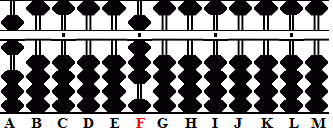```Step 1 A B C D E F G H I J K L M . . .     .   6 0 0 0 0 8 0 0 0 0 0 0 0 ```

Step 2: Multiply 8 x 6 = 48, add the product 48 to rod FG. Notice the technique in this step; 8 on rod F changes to 4 of the product 48.

Determine the new unit rod: The multiplier has one whole number therefore shift one rod to the right of rod F. The new unit rod is rod G leaving the answer 48.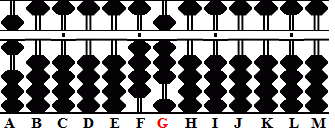```Step 1 A B C D E F G H I J K L M . . .     .   6 0 0 0 0 8 0 0 0 0 0 0 0 + (4)8          7 0 0 0 0 4 8 0 0 0 0 0 0 ```

Example 2: Multiply 78 x 7 = 546

Step 1: Rod F is the unit rod. Set multiplicand 78 on rods EF and multiplier 7 on the left.```Step 1 A B C D E F G H I J K L M . . .     .   7 0 0 0 7 8 0 0 0 0 0 0 0 ```

Step 2: Multiply 7 x 8 = 56, add 56 to rods FG. This step changes 8 on F to 5.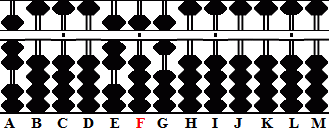```Step 1 A B C D E F G H I J K L M . . .     .   7 0 0 0 7 8 0 0 0 0 0 0 0 + (5)6          7 0 0 0 7 5 6 0 0 0 0 0 0 ```

Step 3: Multiply 7 x 7 = 49; change 7 on E to 4, add 9 to F.

Determine the new unit rod: The multiplier has one whole number therefore shift one rod to the right of rod F. The new unit rod is rod G leaving the answer 546.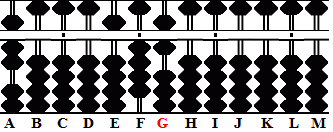```Step 1 A B C D E F G H I J K L M . . .     .   7 0 0 0 7 5 6 0 0 0 0 0 0 + (4)9           7 0 0 0 5 4 6 0 0 0 0 0 0 ```

Example 3: Multiply 23 x 45 = 1035

Step 1: Rod I is the unit rod. Set multiplicand 23 on rods HI. Set multiplier 45 on the left.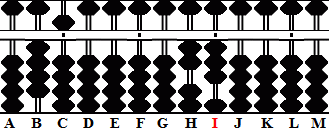```Step 1 A B C D E F G H I J K L M . . .     .   0 4 5 0 0 0 0 2 3 0 0 0 0 ```

Step 2: Multiply 3 x 4 =12, add 12 to rods IJ. For the next step remember that the multiplicand was 3.

2a: Multiply 3 x 5 = 15, add 15 to rods JK. This leave 2 on H and the partial product 135 on rods IJK.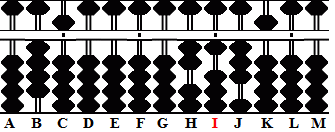```Step 2 A B C D E F G H I J K L M . . .     .   0 4 5 0 0 0 0 2 3 0 0 0 0 + (1)2 Step 2 + 1 5 Step 2a 0 4 5 0 0 0 0 2 1 3 5 0 0```

Step 3: Multiply 2 x 4 = 8, add 08 on rods HI. For the next step remember that the multiplicand was 2.

3a: Multiply 2 x 5 = 10, add 10 to rods IJ leaving the 1035 on rods HIJK.

Determine the new unit rod: The multiplier has two whole numbers therefore shift two rods to the right of rod I. The new unit rod is rod K leaving the answer 1035.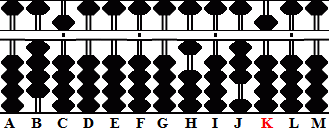```Step 3 A B C D E F G H I J K L M . . .     .   0 4 5 0 0 0 0 2 1 3 5 0 0 + (0)8 Step 3 + 1 0 Step 3a 0 4 5 0 0 0 0 1 0 3 5 0 0 ```

Example 4: Multiply 0.0756 x 0.87 = 0.065772

Step 1: Rod F is the unit rod. Set multiplicand 756 on rods HIJ. Remembering that the multiplier is 0.87 set 87 on the left.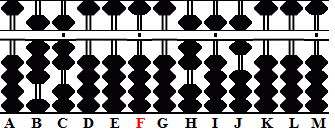```Step 1 A B C D E F G H I J K L M . . .     .   0 0 0 8 7 0 0 7 5 6 0 0 0 ```

Step 2: Multiply 6 x 8= 48, add 48 to rods JK. For the next step remember that the multiplicand was 6.

2a: Multiply 6 x 7 = 42, add 42 to rods KL. This leave 75 on HI and the partial product 522 on rods JKL.```Step 2 A B C D E F G H I J K L M . . .     .   0 0 0 8 7 0 0 7 5 6 0 0 0 + (4)8 Step 2 + 4 2 Step 2a 0 0 0 8 7 0 0 7 5 5 2 2 0```

Step 3: Multiply 5 x  8 = 40, add 40 on rods IJ. For the next step remember that the multiplicand was 5.

3a: Multiply 5 x 7, add 35 to rods JK. This leaves 7 on H and partial product 4872 on rods IJKL.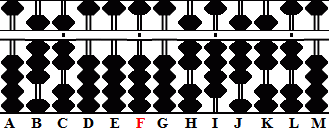```Step 3 A B C D E F G H I J K L M . . .     .   0 0 0 8 7 0 0 7 5 5 2 2 0 +(4)0 Step 3 + 3 5 Step 3a 0 0 0 4 5 0 0 7 4 8 7 2 0```

Step 4: Multiply 7 x 8 = 57, add 56 to HI. For the next step remember that the multiplicand was 7.

4a: Multiply 7 by 7, add the product 49 to rods IJ.

Determine the new unit rod: The multiplier has neither whole numbers nor trailing zeros. The unit rod does not move. Rod F remains the unit rod leaving the answer 0.065772.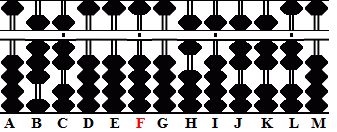```Step 4 A B C D E F G H I J K L M . . .     .   0 0 0 4 5 0 0 7 4 8 7 2 0 +(5)6 Step 4 + 4 9 Step 4a 0 0 0 4 5 0 0 6 5 7 7 2 0```

Example 5: Multiply 486 x 0.0075 = 3.645

Step 1: Rod I is the unit rod. Set multiplicand 486 on rods GHI. Remembering that the multiplier is 0.0075 set 75 on the left.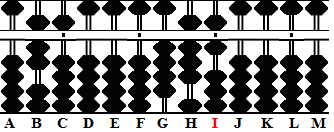```Step 1 A B C D E F G H I J K L M . . .     .   0 7 5 0 0 0 4 8 6 0 0 0 0 ```

Step 2: Multiply 6 x 7 = 42, add 42 to rods IJ. For the next step remember that the multiplicand was 6.

2a: Multiply 6 x 5 = 30, add 30 to rods JK. This leave 48 on rods GH and the partial product 45 on rods IJ.```Step 2 A B C D E F G H I J K L M . . .     .   0 7 5 0 0 0 4 8 6 0 0 0 0 + (4)2 Step 2 + 3 0 Step 2a 0 7 5 0 0 0 4 8 4 5 0 0 0 ```

Step 3: Multiply 8 x 7 56, add 56 to rods HI. For the next step remember that the multiplicand was 8.

3a: Multiply 8 x 5 = 40, add 40 to rods IJ. This leaves 4 on rod G and the partial product 645 on rods HIJ.```Step 3 A B C D E F G H I J K L M . . .     .   0 7 5 0 0 0 4 8 4 5 0 0 0 + (5)6 Step 3 + 4 0 Step 3a 0 7 5 0 0 0 4 6 4 5 0 0 0```

Step 4: Multiply 4 x 7 = 28, add 28 to rods GH. For the next step remember that the multiplicand was 4.

4a: Multiply 4 x 5 = 20,  add 20 to rods HI.

Determine the new unit rod: The multiplier has two trailing zeros after the decimal point. Count two rods to the left of rod I. Rod G is the new unit rod leaving the answer 3.645 on rods GHIJ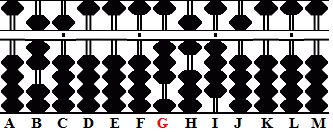```Step 4 A B C D E F G H I J K L M . . .     .   0 7 5 0 0 0 4 6 4 5 0 0 0 + (2)8 Step 4 + 2 0 Step 4a 0 7 5 0 0 0 3 6 4 5 0 0 0 ```

Print Page (.pdf format,127kb)